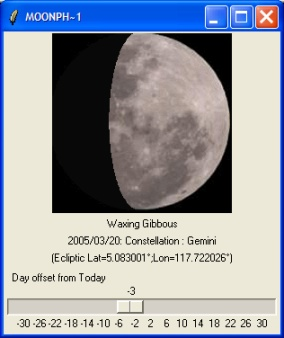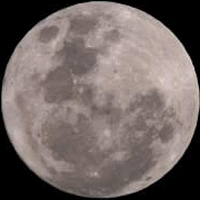## moon phase

Richard Suchenwirth 2004-09-13 - http://www.faqs.org/faqs/astronomy/faq/part3/ (item C.11) describes John Horton Conway's approximation for the moon phase of a given date. Here it is in Tcl:

``` proc moonphase date {
set y [clock format \$date -format %Y]
set delta [expr {\$y/100==19? 4: 8.3}]
scan [clock format \$date -format %m] %d m
set m [expr {\$m==1? 3: \$m==2? 4: \$m}]
scan [clock format \$date -format %d] %d d
set t [expr (\$y%100)%19]
if {\$t>9} {incr t -19}
expr {(round((\$t*11)%30+\$m+\$d-\$delta)%30)}
}```

--- Testing:

``` % moonphase [clock scan "June 6, 1944"]
14 ```

which matches the FAQ's example and stands for (almost) full moon.

Fred Limouzin - 2005-03-21, updated on 2005-03-24: Here's a little week-end project related to the above. It's still a draft, but it's getting there! It simply displays the moon phase in a canvas, based on a percentage value representing the progression within a full moon cycle. 0% is a new Moon, 50% a full Moon, 100% is the next new Moon (in fact 100% is clamped back to 0, etc.).

Here's a screeshot:You'll also need the background image (in fact the most important part!): (I haven't decided yet if the image should be rotated. Last night was overcast ;-))The code and images can also be found at http://dire.straits.free.fr/vertigo ([L1 ]).

``` #!/bin/sh
exec tclsh "\$0" \${1+"\$@"}

package require Tk

set ::OFFSET 99
set ::STEPY  2
set ::pi [expr {acos(-1.0)}]

####################################################
# x^2/a^2 + y^2/b^2 = 1
####################################################
proc ElipseMask {rx1 rx2 ry clr} {
if {\$ry == 0} { return 0 }
set lst [list]
foreach rx [list \$rx1 \$rx2] rysign {1.0 -1.0} {
if {\$rx < 0} {
set rxsign -1.0
set rx [expr {abs(\$rx)}]
} else {
set rxsign 1.0
}
for {set y -\$ry} {\$y <= \$ry} {incr y \$::STEPY} {
set t [expr {1.0 - ((1.0 * \$y * \$y) / (1.0 * \$ry * \$ry))}]
set x [expr {round(1.0 * \$rx * sqrt(\$t))}]
lappend lst [expr {round((\$rxsign * \$x) + \$::OFFSET)}]
lappend lst [expr {round((\$rysign * \$y) + \$::OFFSET)}]
}
}
return [.c create polygon \$lst -fill \$clr -outline \$clr]
}

####################################################
# p in percent of a full cycle within [0.0;100.0]
# 0% and 100% : new moon; 50% full moon; etc.
####################################################
# This gives an approximation for a flat Moon rather
# than spherical Moon. But after all it is well known
# that Earth is flat, so the Moon must be too...
####################################################
proc ElipsePhase {p} {
while {\$p >= 100.0} {
set p [expr {1.0 * (\$p - 100.0)}]
}
set phasesLst { {New Moon} {Waxing Crescent} {First Quarter}  \
{Waxing Gibbous} {Full Moon} {Waning Gibbous} \
{Last Quarter} {Waning Crescent} }
set idx [expr {int(8.0 * (\$p + (100.0/16.0)) / 100.0) % 8}]
set phase [lindex \$phasesLst \$idx]
set quadrant [expr {int(1.0 * \$p / 25.0)}]
set mod [expr {(1.0 * \$p) - (25.0 * \$quadrant)}]
set rx1 [expr {-1.0 * (4.0 * \$mod)}]
} elseif {\$quadrant == 2} {
set rx1 [expr {100.0 - (4.0 * \$mod)}]
} else {
}
set rx2 [expr {100.0 - (4.0 * \$mod)}]
} elseif {\$quadrant == 1} {
set rx2 [expr {-1.0 * (4.0 * \$mod)}]
} else {
}
return [list \$rx1 \$rx2 \$phase]
}

# normalize in [0.0;1.0]
proc normalize {{v 0.0}} {
set v [expr {1.0 * (\$v - floor(\$v))}]
if {\$v < 0.0} {
set v [expr {1.0 + \$v}]
}
return \$v
}

# based on "Lunar Phase Computation" by Stephen R. Schmitt
# based on "Sky & Telescope, Astronomical Computing", April 1994
proc ComputeLunarPhase {{tmr {}}} {
if {\$tmr eq {}} {
set tmr [clock seconds] ;# today by default
}
set year [clock format \$tmr -format %Y]
scan [clock format \$tmr -format %m] %d month
scan [clock format \$tmr -format %d] %d day
set date [format {%4d/%02d/%02d} \$year \$month \$day]
# Julian date at 12h UT
set JulianYear [expr {\$year - int((12.0 - \$month) / 10.0)}]
set JulianMonth [expr {(\$month + 9) % 12}]
set K1 [expr {int(365.25 * (\$JulianYear + 4712.0))}]
set K2 [expr {int((30.6 * \$JulianMonth) + 0.5)}]
set K3 [expr {int(floor((\$JulianYear / 100.0) + 49.0) * 3 / 4.0) - 38}]
set JulianDay [expr {\$K1 + \$K2 + \$day + 59}]
set GregorianDay [expr {\$JulianDay - \$K3}]
set JGDay [expr {(\$JulianDay > 2299160) ? \$GregorianDay : \$JulianDay}]
#calculate moon's age in days
set MaxAge 29.530588853
set IP [normalize [expr {(\$JGDay - 2451550.1) / \$MaxAge}]]
set MoonAge [expr {\$IP * \$MaxAge}]
set pc [expr {(100.0 * \$MoonAge) / \$MaxAge}]
#calculate moon's distance
set tmp [normalize [expr {(\$JGDay - 2451562.2 ) / 27.55454988}]]
set DP [expr {2.0 * \$::pi * \$tmp}]
set dist [expr {60.4 - 3.3*cos(\$DP) - 0.6*cos((2*\$IPrad) - \$DP)}]
set dist [expr {\$dist - 0.5*cos(2*\$IPrad)}]
#calculate moon's ecliptic latitude
set tmp [normalize [expr {(\$JGDay - 2451565.2) / 27.212220817}]]
set NP [expr {2.0 * \$::pi * \$tmp}]
set EclipticLatitude [expr {5.1 * sin(\$NP)}]
# calculate moon's ecliptic longitude
set RP [normalize  [expr {(\$JGDay - 2451555.8) / 27.321582241}]]
set tmp [expr {(360.0 * \$RP) + (6.3*sin(\$DP))}]
set tmp [expr {\$tmp + (1.3*sin(2.0*\$IPrad - \$DP))}]
set EclipticLongitude [expr {\$tmp + (0.7*sin(2.0*\$IPrad))}]

if       {\$EclipticLongitude <  33.18} {    set Zodiac "Pisces"
} elseif {\$EclipticLongitude <  51.16} {    set Zodiac "Aries"
} elseif {\$EclipticLongitude <  93.44} {    set Zodiac "Taurus"
} elseif {\$EclipticLongitude < 119.48} {    set Zodiac "Gemini"
} elseif {\$EclipticLongitude < 135.30} {    set Zodiac "Cancer"
} elseif {\$EclipticLongitude < 173.34} {    set Zodiac "Leo"
} elseif {\$EclipticLongitude < 224.17} {    set Zodiac "Virgo"
} elseif {\$EclipticLongitude < 242.57} {    set Zodiac "Libra"
} elseif {\$EclipticLongitude < 271.26} {    set Zodiac "Scorpio"
} elseif {\$EclipticLongitude < 302.49} {    set Zodiac "Sagittarius"
} elseif {\$EclipticLongitude < 311.72} {    set Zodiac "Capricorn"
} elseif {\$EclipticLongitude < 348.58} {    set Zodiac "Aquarius"
} else {                                    set Zodiac "Pisces"
}
return [list \$pc \$date \$dist \$EclipticLatitude \$EclipticLongitude \$Zodiac]
}

####################################################
proc UpdateMoon args {
global obj
global phaselbl
global infotxt
global zodiac

set secinaday [expr {24 * 60 * 60}]
set co  #080808
catch {.c delete \$obj} res
set dayoffset [.s get]
set tmr [expr {[clock second] + (\$dayoffset * \$secinaday)}]
;#;#test: 28 fev 2004 new moon, 6 march 2004 full, 13 march last quarter
;#set tmr [expr {[clock scan {March 1, 2004}] + (\$dayoffset * \$secinaday)}]
foreach {pc date -- eclat eclon zod} [ComputeLunarPhase \$tmr] {break;#}
set infotxt [format {(Ecliptic Lat=%f°;Lon=%f°)} \$eclat \$eclon]
set zodiac [format {%s: Constellation : %s} \$date \$zod]
foreach {rx1 rx2 phaselbl} [ElipsePhase \$pc] {break;#} ;#assign
set obj [ElipseMask \$rx1 \$rx2 \$ry \$co]
update
}

####################################################
set phaselbl {}
set zodiac   {}
set infotxt  {}
canvas .c -width [expr {2 * \$::RADIUS}] \
-height [expr {2 * \$::RADIUS}] -background black
label .l -textvariable phaselbl
label .i -textvariable infotxt
label .z -textvariable zodiac
scale .s -from -30 -to 30 -length 300 -resolution 1    \
-label "Day offset from Today" -variable dayoffset -command {UpdateMoon} \
-orient horizontal -tickinterval 4 -showvalue true

pack .c -side top
pack .s -side bottom
pack .i -side bottom
pack .z -side bottom
pack .l -side bottom

# Full moon image as the 'background'
set fname [file join [file dirname [info script]] moon.gif]
set img [image create photo -file \$fname]
.c create image 0 0 -image \$img -anchor nw